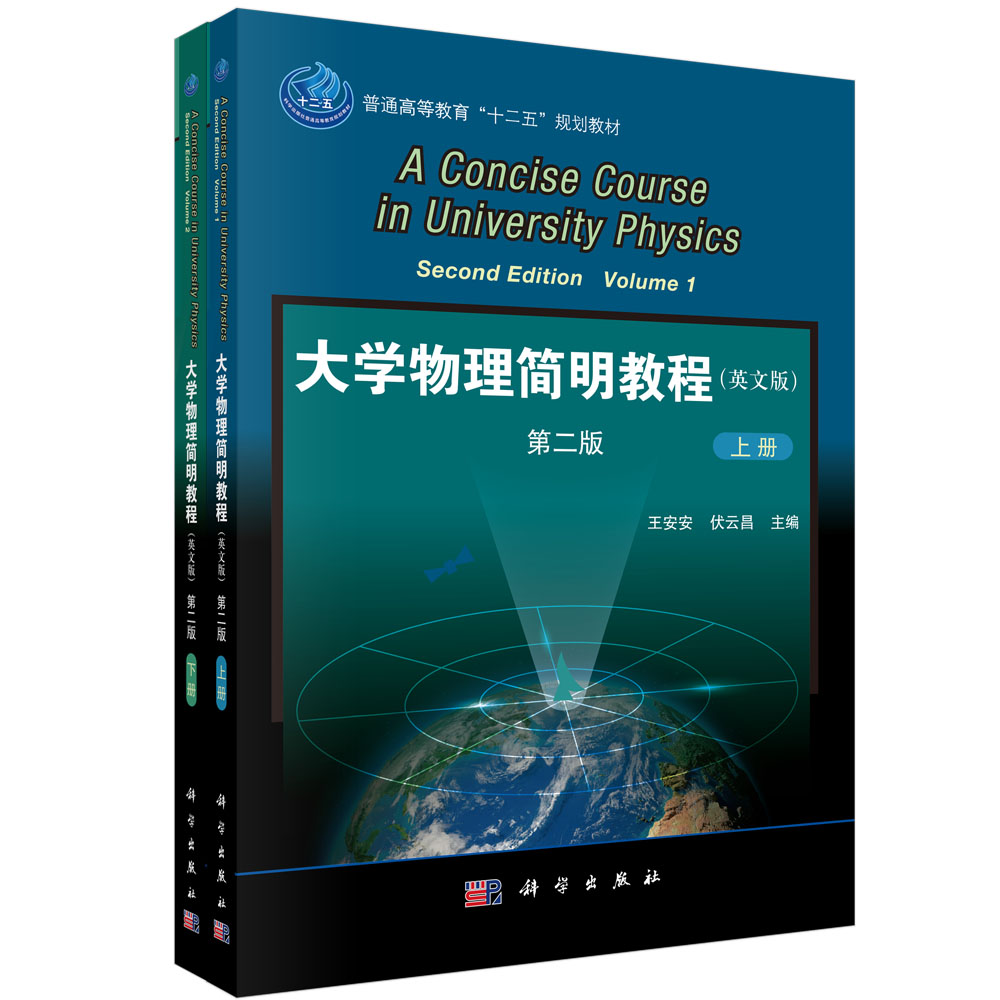0去购物车结算

### 浏览历史• 书号：9787030365545
作者：王安安,伏云昌
• 外文书名：A Concise Course in Universit Physics Second Edition
• 装帧：平装
开本：16
• 页数：249
字数：784000
语种：zh-Hant
• 出版社：科学出版社
出版时间：2013-01-01
• 所属分类：O4 物理学 0702 物理学
• 定价： ￥79.00元
售价： ￥63.20元
• 图书介质:
纸质书 电子书• 购买数量： 件  可供
• 商品总价：
•• 暂时还没有任何用户评论

### 全部咨询(共0条问答)

• 暂时还没有任何用户咨询内容

 用户名： 匿名用户 E-mail： 咨询内容：

### 目录

• Contents
PartOne Mechanics
Chapter1 Kinematics 2
1.1 Frame of Referenceand Particle 2
1.2 Displacement,Velocity,and Acceleration 4
1.3 Two Types of Problems in Kinematics and Sample Problems 10
1.4 Circular Motion 13
1.5 Relative Motion 17
Questions 19
Problems 20
Chapter2 Dynamics—Newton's Laws of Motion 23
2.1 Newton's Laws,Force,and Inertial Reference Frame 23
2.2 The Forces in Mechanics and the Fundamental Forces in Nature 27
2.3 Fundamental Quantities,Units,and Dimensions 33
2.4 Applying Newton's Laws of Motion 34
2.5 Galilean Transformations 39
2.6 Non-inertial Frame and Inertial Force 40
Questions 44
Problems 45
Chapter3 Work and Energy 49
3.1 Work 49
3.2 Kinetic Energy and the Law of Kinetic Energy 54
3.3 Conservative Force and Potential Energy of Weight 55
3.4 Elastic Potential Energy and Universal Gravitational Potential Energy 58
3.5 Conservation of Mechanical Energy 59
3.6 The Conservation of Energy 64
Questions 65
Problems 66
Chapter4 Momentum 70
4.1 Linear Momentum,Linear Impulse,and Momentum Theorem 70
4.2 Conservation of Momentum 73
4.3 Collision 76
4.4 Conservation of Angular Momentum of a Particle 80
4.5 The Center of Mass 83
Questions 86
Problems 87
Chapter5 Rotation of a Rigid Body 92
5.1 Motion of a Rigid Body 92
5.2 Torque,the Law of Rotation,and Rotational Inertia 94
5.3 Applying the Law of Rotation 99
5.4 Kinetic Energy and Work in Rotational Motion 101
5.5 Angular Momentum of a Rigid Body and Conservation of Angular Momentum 104
Questions 109
Problems 110
PartTwo Electromagnetism
Chapter6 Static Electric Field in a Vacuum 116
6.1 Electric Charge and Coulomb's Law 116
6.2 The Electric Field 118
6.3 Electric Field Lines,Electric Flux,and Gauss'Law 123
6.4 Electric Potential 130
6.5 Equipotential Surface and Potential Gradient 135
6.6 The Electric Force Exerted on a Moving Charged Particle 136
Questions 138
Problems 139
Chapter7 Conductors and Dielectrics in Electrostatic Field 143
7.1 Conductors and Electrostatic Induction 143
7.2 Capacitors and Capacitance 147
7.3 Dielectrics 152
7.4 Gauss'Law in Dielectric 155
7.5 Energy Stored in anElectric Field 159
Questions 161
Problems 162
Chapter8 Magnetic Field of a Steady Current 165
8.1 The Magnetic Phenomena and Ampere's Hypothesis 165
8.2 The Magnetic Field,Magnetic Field Lines,and Magnetic Flux 167
8.3 Magnetic Fields Set Up by a Current and a Moving Charge 169
8.4 Ampere's Law 173
8.5 Motion of a Charged Particle in a Magnetic Field 176
8.6 Magnetic Force on Current-carrying Conductors 178
8.7 Magnetic Torque on a Current-carrying Loop 179
*8.8 The Hall Effect 180
8.9 Magnetic Material 181
8.10 Ampere's Law and Gauss'Law for Magnetism 185
*8.11 Ferromagnetism 188
Questions 193
Problems 194
Chapter9 Electromagnetic Induction 199
9.1 Nonelectrostatic Force,Source and Electromotive Force 199
9.2 Faraday's Law of Induction 202
9.3 Motional Electromotive Force 206
9.4 Induced Electric Fields 209
9.5 Self-induction and Mutual-induction Phenomena 212
9.6 Energy of the Magnetic Field 216
9.7 Displacement Current and Complete Current Law 218
9.8 Maxwell's Equations 223
Questions 226
Problems 228
Appendix1 The International System of Units 234
Appendix2 Some Fundamental Constants of Physics 236
Words 237